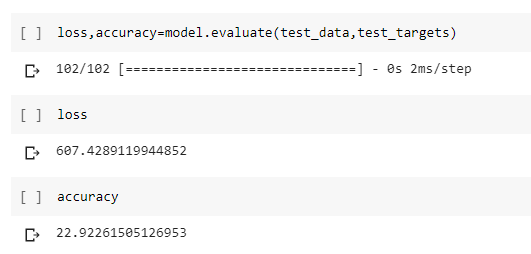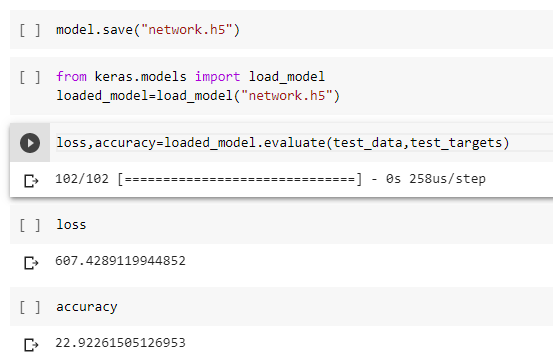# ML – Saving a Deep Learning model in Keras

Training a neural network/deep learning model usually takes a lot of time, particularly if the hardware capacity of the system doesn’t match up to the requirement. Once the training is done, we save the model to a file. To reuse the model at a later point of time to make predictions, we load the saved model.
Through Keras, models can be saved in three formats:

• YAML format
• JSON format
• HDF5 format

YAML and JSON files store only model structure, whereas, HDF5 file stores complete neural network model along with structure and weights. Therefore, if the model structure is saved using YAML or JSON format, weights should be stored in an HDF5 file to store the entire model.
Considering Boston house prices dataset:

 `import` `keras ` `from` `keras.datasets ``import` `boston_housing ` ` `  `(train_data, train_targets), (test_data, test_targets)``=` `boston_housing.load_data() ` ` `  `mean ``=` `train_data.mean(axis ``=` `0``) ` `train_data``-``=` `mean ` `std ``=` `train_data.std(axis ``=` `0``) ` ` `  `train_data``/``=` `std ` `test_data``-``=` `mean ` `test_data``/``=` `std `

Code: Training a neural network model on it

 `from` `keras ``import` `models ` `from` `keras ``import` `layers ` ` `  `model ``=` `models.Sequential() ` `model.add(layers.Dense(``64``, activation ``=``"relu"``, input_shape ``=``(train_data.shape[``1``], ))) ` `model.add(layers.Dense(``64``, activation ``=``"relu"``)) ` `model.add(layers.Dense(``1``)) ` `model.``compile``(optimizer ``=``"rmsprop"``, loss ``=``"mse"``, metrics ``=``[``"mae"``]) ` `loss, accuracy ``=` `model.evaluate(test_data, test_targets) `

Output:`from` `keras.models ``import` `load_model ` `model.save(``"network.h5"``) ` `loaded_model ``=` `load_model(``"network.h5"``) ` `loss, accuracy ``=` `loaded_model.evaluate(test_data, test_targets) `

Output:`# Saving model structure to a JSON file ` ` `  `model_json ``=` `model.to_json() ``# with open("network.json", "w") as json_file: ` `    ``json_file.write(model_json) ` ` `  `# Saving weights of the model to a HDF5 file ` `model.save_weights(``"network.h5"``) ` ` `  `# Loading JSON file  ` `json_file ``=` `open``(``"network.json"``, ``'r'``) ` `loaded_model_json ``=` `json_file.read() ` `json_file.close() ` `loaded_model ``=` `model_from_json(loaded_model_json) ` ` `  `# Loading weights ` `loaded_model.load_weights(``"network.h5"``) ` `loss, accuracy ``=` `loaded_model.evaluate(test_data, test_targets) `

 `# Saving model structure to a YAML file ` `model_yaml ``=` `model.to_yaml()  ` `with ``open``(``"network.yaml"``, ``"w"``) as yaml_file: ` `    ``yaml_file.write(model_yaml) ` ` `  `# Saving weights of the model to a HDF5 file ` `model.save_weights(``"network.h5"``) ` ` `  `# Loading YAML file  ` `yaml_file ``=` `open``(``"network.yaml"``, ``'r'``) ` `loaded_model_yaml ``=` `yaml_file.read() ` `yaml_file.close() ` `loaded_model ``=` `model_from_yaml(loaded_model_yaml) ` ` `  `# Loading weights ` `loaded_model.load_weights(``"network.h5"``) ` `loss, accuracy ``=` `loaded_model.evaluate(test_data, test_targets) `

My Personal Notes arrow_drop_upCheck out this Author's contributed articles.

If you like GeeksforGeeks and would like to contribute, you can also write an article using contribute.geeksforgeeks.org or mail your article to contribute@geeksforgeeks.org. See your article appearing on the GeeksforGeeks main page and help other Geeks.

Please Improve this article if you find anything incorrect by clicking on the "Improve Article" button below.